New update is available. Click here to update.

# Find elements which are present in one array but not in other

## Introduction

You are given two arrays in the task "Find elements which are present in the first array and not in the second." All the integers are contained in arrays. You must identify the integers that will appear in the first array but not the second array.Example :

Array 1

Array 2

The elements which are in red colour in array1 are the elements which are not present in the second array.

### 1st Method : Simple

Using two loops and checking for elements that are missing from the second array is a simple approach.

To understand this approach you need to follow these simple steps

Step 1 : Start the first for loop from index i=0 to n-1 length as shown in the figure.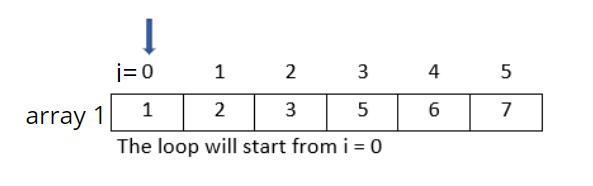Step 2 : Now compare the zeroth element of the array 1 to all the elements of the second array.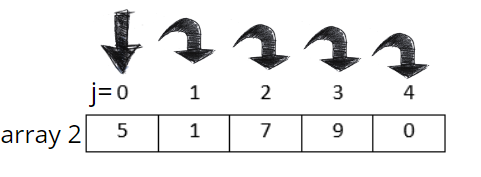Step 3: If  arry1 =arry2 (i.e arry1[i]=arr[j]) it will break the loop there only else j will be incremented and it will compare arry1[i] to arry2[j].

Step 4: If the element of the first array is not present in the second array it will print that number only.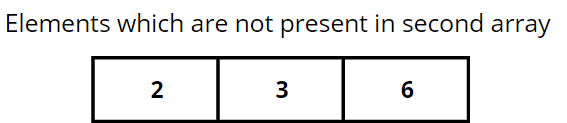### Implementation

``````#include<bits/stdc++.h>
using namespace std;

// Function to find elements which are present  in arry1[]  but not present in arry2[].

void Missingnum(int arry1[], int arry2[],
int n, int m)
{
for (int i = 0; i < n; i++)
{
int j;
for (j = 0; j < m; j++)
if (arry1[i] == arry2[j])
break;

if (j == m)
cout << arry1[i] << " ";
}
}

// Driver code
int main()
{
int arry1[] = { 1, 2, 3, 5, 6, 8 };
int arry2[] = { 5, 1, 7, 9, 0 };
int n = sizeof(arry1) / sizeof(arry1);
int m = sizeof(arry2) / sizeof(arry2);
Missingnum(arry1, arry2, n, m);
return 0;
}``````

### Output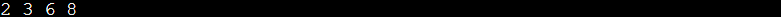Using inner and outer loops, the time complexity is O(n*m) and Auxiliary space is O(1).

### 2nd Method : Use Hashing

With this method, the second array's elements are all stored in an unordered set hash table. All the elements in the first array should be checked one by one, and those that are missing from the hash table should be printed. With hashmap, the search complexity will be O(1) which is way faster.

Refer to below pictorial representation to get an idea about the intuition behind this approach.

### Implementation in C++

``````#include<bits/stdc++.h>
using namespace std;

/* Function to find elements which are present in arry1[]  but not present in arry2[].*/

void Missingnum(int arry1[], int arry2[],
int n, int m)
{
/*Storing all elements of second array*/
unordered_set <int> s;
for (int i = 0; i < m; i++)
s.insert(arry2[i]);

/* Printing all the  elements of arry1 that are not present in hash table*/
for (int i = 0; i < n; i++)
if (s.find(arry1[i]) == s.end())
cout << arry1[i] << " ";
}

/* Driver code*/
int main()
{
int arry1[] = { 1, 2, 5, 8, 9, 0 };
int arry2[] = { 2, 5, 6, 7, 0 };
int n = sizeof(arry1) / sizeof(arry1);
int m = sizeof(arry2) / sizeof(arry2);
Missingnum(arry1, arry2, n, m);
return 0;
}
``````

### Output: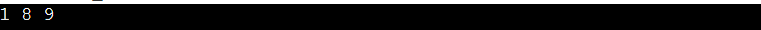Using inner and outer loops, the time complexity is O(n+m) and Auxiliary space is O(n)

### Implementation in Java

``````/* Java efficient program to find element  which are not present in second array*/

import java.util.HashSet;
import java.util.Set;

public class Main {

/* Function for finding elements which are there in x[] but not in y[].*/
static void findMissing(int x[], int y[],
int n, int m) {
/* Store all elements of second array in a hash table*/
HashSet < Integer > s = new HashSet < > ();
for (int i = 0; i < m; i++)

/* Print all elements of first array  that are not present in hash table*/
for (int i = 0; i < n; i++)
if (!s.contains(x[i]))
System.out.print(x[i] + " ");
}

public static void main(String[] args) {

int x[] = { 2, 3, 7, 9, 4, 1 };
int y[] = { 2, 4,6,4,0};
int n = x.length;
int m = y.length;
findMissing(x, y, n, m);
}
}
``````

### Output: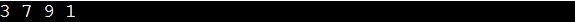Using inner and outer loops, the time complexity is O(n+m) and Auxiliary space is O(n)

### How can you determine whether an element in one array is also in another?

To determine whether an array has every element in another array we need to follow these simple steps: first sort both the given arrays, second iterate over both the arrays simultaneously, lastly at a given iteration if the value of both the iterators are different, return the element from the first array, as it is the missing element.

### In C++, how can I find unique elements in an array?

There are two methods for finding the non-repeating element in an array : Method 1 is use two loops, one to check whether the element is already present in the array or not and the other to check for the current element and method 2 is iterate through the array and add each element's frequency in the hash table.

### Using XOR, how can one identify unique elements in an array?

The XOR bitwise operation, which only returns 1 if one of the elements is 1 and false otherwise, can be used to identify the unique element in which each integer in the array is XORed with uniqueId starting at 0 during the loop.

### How do we store data in a hashmap?

In hashmap data is stored in the form of the key value pair it means every key will have some  value or data mapped to it.

### How do we compare one array with another?

To compare two arrays, we must ensure that their lengths are the same, that the objects they contain are of the same type, and that each element in one array is equivalent to its counterpart in the other array and by doing this, we can determine whether or not the two arrays are similar.

## Conclusion

To summarize this blog, we came across two different approaches and examples to find elements which are present in one array but not in other.To enhance your understanding you can refer to these articles: introduction-to-hashing, string-hashing implementation-of-hashmap,double-hashing,difference-between-hashmap-and-hashtable and many more

Refer to our guided paths on Codestudio to learn more about DSA, Competitive Programming, JavaScript, System Design, etc. Enroll in our courses and refer to the mock test and problems available, Take a look at the interview experiences and interview bundle for placement preparations.

Do upvote our blog to help other ninjas grow.

Happy Learning!